Precalculus : Sum and Difference Identities For Cosine

Example Questions

Example Question #1 : Sum And Difference Identities For Cosine

Evaluate the exact value of: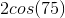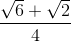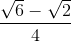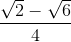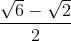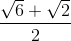Explanation:

In order to solve, two special angles will need to be used to solve for the exact values.

The angles chosen are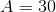and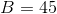degrees, since: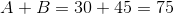Write the formula for the cosine additive identity.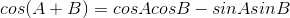Substitute the known variables.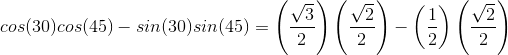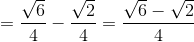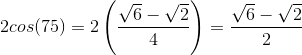Example Question #2 : Sum And Difference Identities For Cosine

Find the exact value of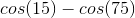.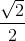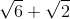Explanation:

To solve, we will need to use both the sum and difference identities for cosine.

Write the formula for these identities.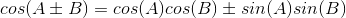To solve for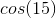and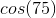, find two special angles whose difference  and sum equals to the angle 15 and 75, respectively.  The two special angles are 45 and 30.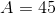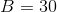Substitute the special angles in the formula.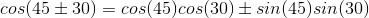Evaluate both conditions.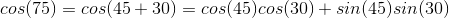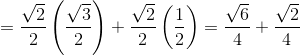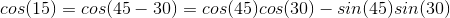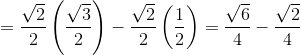Solve for.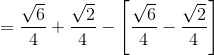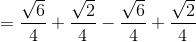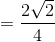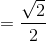Example Question #3 : Sum And Difference Identities For Cosine

In the problem below,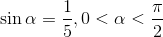and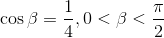.

Find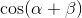.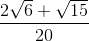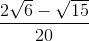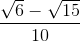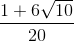Explanation:

Since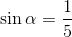and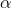is in quadrant I, we can say that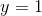and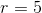and therefore: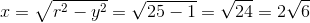So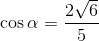.

Since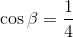and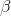is in quadrant I, we can say thatand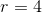and therefore: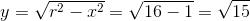.  So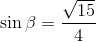.

Using the cosine sum formula, we then see: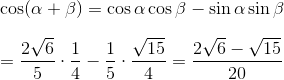.

Example Question #4 : Sum And Difference Identities For Cosine

In the problem below,and.

Find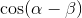.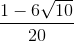Explanation:

Sinceandis in quadrant I, we can say thatandand therefore:So.

Sinceandis in quadrant I, we can say thatandand therefore:So.

Using the cosine difference formula, we see: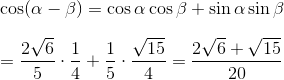All Precalculus Resources